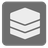Create Intervals
Simple
English RU

From a set of ints you have to create a list of closed intervals as tuples, so the intervals are covering all the values found in the set.

A closed interval includes its endpoints! The interval 1..5, for example, includes each value x that satifies the condition 1 <= x <= 5.

Values can only be in...
A single value, that does not fit into an existing interval becomes the start- and endpoint of a new interval.

A set of ints.

A list of tuples of two ints, indicating the endpoints of the interval. The Array should be sorted by start point of each interval

```create_intervals({1, 2, 3, 4, 5, 7, 8, 12}) == [(1, 5), (7, 8), (12, 12)]
create_intervals({1, 2, 3, 6, 7, 8, 4, 5}) == [(1, 8)]```
You should be an authorized user in order to see the full description and start solving this mission.